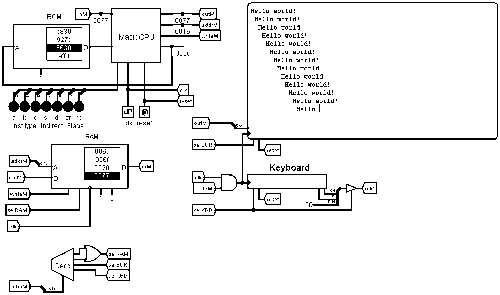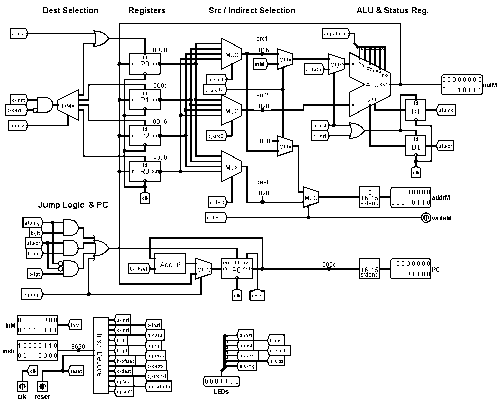# Ideas for a Slightly More Powerful CPUClassicListThreaded7 messagesOpen this post in threaded view
|

## Ideas for a Slightly More Powerful CPU

Open this post in threaded view
|

## Re: Ideas for a Slightly More Powerful CPU

 I found a bug. The general Hack computer architecture has only one address bus from CPU to memory, used to both identify the location of the data to fetch from RAM and write back to RAM. Therefore, the CPU cannot read from one address and write back to a different address in an instruction. This means my CPU cannot do, for example, *R3 = *R2 + 1, i.e. load from the address which R2 points to, and write back to the address in R3. So, the solution is, when the i bit is set in both idd and iss, the src1 value (i.e the ss in iss) is replaced with the destination value. This allow the CPU to do *R3 = *R3 + 1.
Open this post in threaded view
|

## Re: Ideas for a Slightly More Powerful CPU

For a lunch time back-of-the-envelope doodle, this is quite a clever design.

Implementing this architecture in LogiSim has been on my to do list for quite a while and I finally got around to it.Note: type in the keyboard while the program is running to change the message.

 Files Mack.circ LogiSim circuit MackHello.lsbin Hello world program (if you need to reload the ROM) MackHello.lst Commented source

Along the way I discovered some interesting details.

## Easily extended Jump instruction

As I was wiring up the PC load address for the Jump instruction (Branch with jjj = 0) I had a rather snaky wire running its way to R0. It went past the ALU on it way and I got to thinking...

The Jump instruction, 11 000 xxxxxxxxxxx, has all the correct bits available in the undefined region to turn it into a PC= instruction with full ALU computation including indirect src1.
11 000 iss oooooo ss

This may be quite useful for return IPs for assembly language subroutines, computed jumps and implementation of call and return stack functions. It appears useful if the PC= instruction does not set the status bits; there may be a way to use the zr flag as a subroutine pass/fail return value.

```        R0 = RIP_1
R3 = R0
BR      Function
RIP_1:
BNE     Error
...

Function:
....
R0 = 0  // good result
PC = R3
```

## Status bits and overloaded R0=n instruction

There is a problem with the above code snippet. Depending on how the assembler encodes the R0=0 instruction, it may be an A-instruction that does not set the zr flag. I changed the data routing for the A-instruction—instead of muxing the data directly into R0, I am muxing it into the ALU x input, setting the ALU function to out=x, and loading the status register.

## More macros

If R0 is considered a scratch register that the assembler my use at will, then additional useful macros are possible. For example:
```    Rd=-6               R0=6; Rd=-R0
*Rd=123,            R0=123; *Rd=R0
Rd=Rs+16, s!=0      R0=16; Rd=Rs+R0
```

## How much do the extra registers help?

Here is an assembly version of C's strcpy. The parameters are pointers to the string data.
On Hack, the parameters and RIP are passed in memory varaibles; on Mack they are passed in registers.
      Hack       Mack ```// call strcpy (dest, src) @src D=M @strcpy_src M=D @dest D=M @strcpy_dest M=D @rip_123 D=A @strcpy_rip M=D @strcpy 0;JMP ... strcpy: @strcpy_src M=M-1 @strcpy_dest M=M-1 loop: @strcpy_src AM=M+1 D=M @p2 AM=M+1 M=D @loop D;JNE @strcpy_rip A=M 0;JMP ``` ```// call strcpy (dest, src) r0=src r1=*r0 r0=dest r2=*r0 r0=rip_123 r3=r0 r0=strcpy // jump strcpy PC=r0 // macro rip123: ... strcpy: r1=r1-1 r2=r2-1 loop: r1=r1+1 r2=r2+1 r0=*r1 *r2=r0 bne loop PC=r3 ```
The Hack call + function runs in 23 + 8n instructions, the Mack call + function in 11 + 5n, where n is the string length including the terminating 0.

--Mark

Open this post in threaded view
|

## Re: Ideas for a Slightly More Powerful CPU

 This post was updated on . In reply to this post by Warren Toomey How does the relative branch work? For example in the code snippet shared: // Sum the Numbers from 1 to 100 // ------------------------------------ 0:    R1 = 1                    // i = 1 1:    R2 = 0                    // sum = 0     loop: 2:    R2 = R2 + R1          // sum = sum + i 3:    R1 = R1 + 1            // i++ 4:    R0 = 100 5:    R0 = R1 - R0           // calculate i - 100 6:    BLE loop                  // loop while i <= 100     end: 7:    JMP end                  // end with infinite loop What would be the 11bit constant used for the BLE? loop is at address 2. The BLE instruction is at address 6. Given that for a branch we "sign extend the constant to 16 bits, and add it to the PC", we would have to add -4 to the PC to get to loop from the BLE instruction. -4 is 65532 which is more than can be represented with 11 bits... Edit: -1 is bin(65535) is 0b1111111111111111 -2 is bin(65534) is 0b1111111111111110 -3 is bin(65533) is 0b1111111111111101 -4 is bin(65532) is 0b1111111111111100 -5 is bin(65531) is 0b1111111111111011 Seems to be a pattern that is somehow useful...?
Open this post in threaded view
|

## Re: Ideas for a Slightly More Powerful CPU

 Also, how would you conditionally branch to a location that is more than 2^11 addresses away from the branch instruction?
 Administrator Sign extension is how shorter 2's complement numbers are converted to longer numbers. Take the sign bit from the shorter number and use it for all the high order bits of the longer number. ``` sxx xxxx xxxx 11-bit 2's complement ssss ssxx xxxx xxxx 16-bit 2's complement ```Conditional jumps to locations more than +/- 1023 away are quite rare because they are primarily only used in conditional and looping control structures. The dependent code in ifs and loops should be relatively small. The only way to code an absolute conditional jump would be to use a relative "skip" around a jump. ``` BNE skip // BEQ far_away JMP far_away skip: ```The assembler would need to flag any conditional jumps that are too far away as errors and the programmer would need to fix them. The assembler can not do this automatically because the absolute jump uses R0, and it cannot know if R0 is safe to use. --Mark